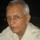Coffee Room
Discuss anything here - everything that you wish to discuss with fellow engineers.
12933 Members
Join this group to post and comment.Why Zero Important?

Today I was listining song "Hae Preet Zahan ki Reet" from Purab aur paschim

there was serious argument between pran and manoj kumar just before that song about India

In this song there are lines "जब ज़ीरो दिया मेरे भारत ने ,भारत ने मेरे भारत ने ,दुनिया को तब गिनती आयी"

Why is zero so much important, what could have happened if zero was not there?Sanyam Khurana • May 24, 2014
Manish Goyal
Today I was listining song "Hae Preet Zahan ki Reet" from Purab aur paschim

there was serious argument between pran and manoj kumar just before that song about India

In this song there are lines "जब ज़ीरो दिया मेरे भारत ने ,भारत ने मेरे भारत ने ,दुनिया को तब गिनती आयी"

Why is zero so much important, what could have happened if zero was not there?
Seems interesting...

Well, if "Zero" was not there, probably we cannot count beyond 9 or just can't start to count, 0 is the first numberAnoop Kumar • May 24, 2014
Because, I am engineer and start counting with 0 ☕

Anyway here you can find some of importance in maths
https://nrich.maths.org/5598
https://mathforum.org/library/drmath/view/57051.html

In simple word, Zero is the thing which adds value to a digit in number.If zero is not there it would be difficult to count beyond 9 because in ancient times they used Roman numerals to count which is more difficult to follow on.
Due to invention of Zero by Aryabatta , the maths revolution started.zaveri • May 25, 2014
If there was no zero, then i guess we all would not be here chatting in CE !😀MDCCCLXXXVIII is a Roman numeral, which has no zero. in Arabic numerals with zero this becomes 1888.

This is important I guess.Ankita Katdare • May 25, 2014
I think Zero is ALSO so important because it helps us count items in large quantities or smaller quantities. Zero (or Shunya in Sanskrit, which means vacuum or non-existential) is the basis of every computation.

1
10
100
1000
10000

or

0
0.1
0.01
0.001
0.0001

When I was a kid, I had a hard time understanding how decimal system worked. And now, I can't even begin to imagine - how deep an impact the "0" makes.ISHAN TOPRE • May 25, 2014
SarathKumar Chandrasekaran
Due to invention of Zero by Aryabatta , the maths revolution started.
Thank you for calling me Aryabhatta. You see, my contribution to maths is also "ZERO". In fact, my contribution in every field is "ZERO".😁

Jokes apart, These are some simple points.

1. we should think of 0 as a concept and not a numeral. For example in electrical zero is ground but is considered as a source of infinite negative potential.

2. In maths 0!=1!=1.

3. In linear Algebra "Zero" is not "Zero". I.e; In any matrix, zero has a meaning of "quantity which is zero".
But if you leave a space blank, it means that there is no character and the variable is absent I.E; non- existent.

3. Zero is also a level. In measuring pressure, Vacuum pressure is considered as 0 bar but atmospheric pressure is measured with reference to sea level.

Height of sea level from sea level- 0 meter.
But pressure at sea level= 1.01325 Bar.

4. Zero is taken as base, in temperature measurement. It is referenced to many different points like triple point, boiling point, freezing point etc.

5. In logarithm however, the base is 10. For calculating natural log, the base is 'e'=2.718.